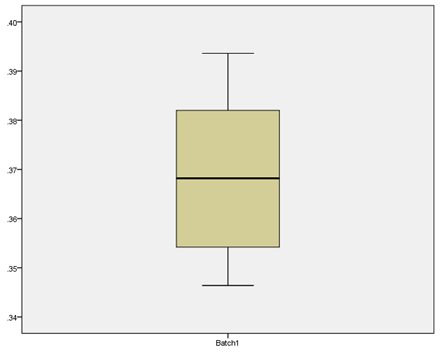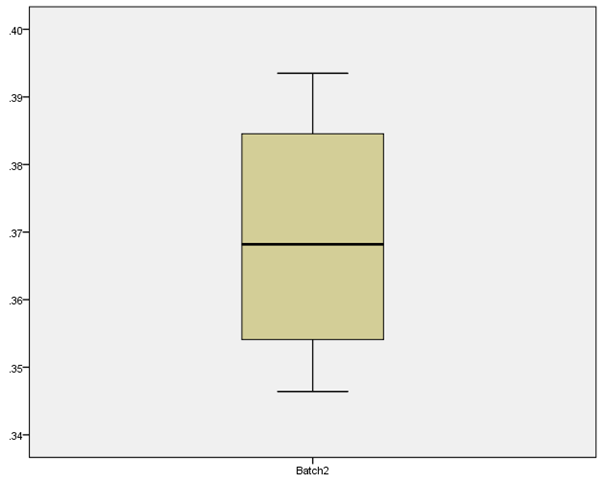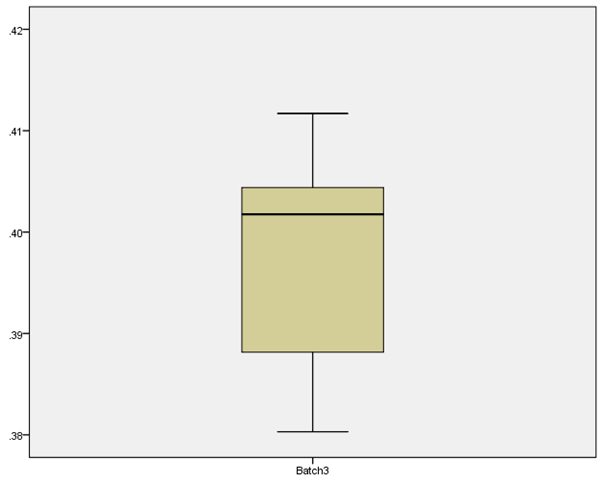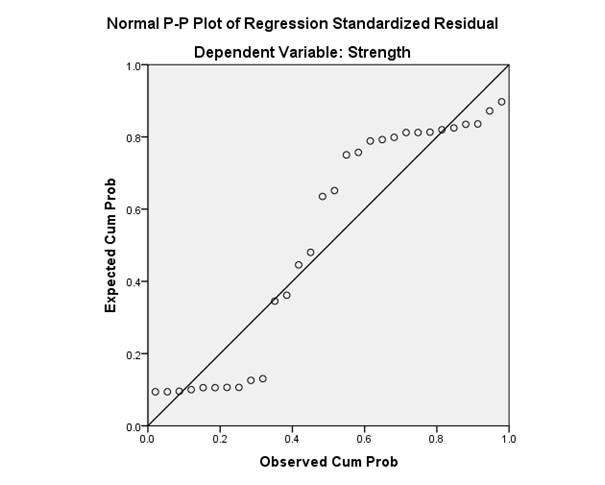# Pharmaceutics Assignment-MAH_130115_25370_2_49566

We have to compare the three batches in terms of their arithmetic mean tablet weight. For this comparison purpose, we have to see some descriptive statistics for these three batches. We have to use the one way ANOVA test for comparison of means of three batches. We have to use the SPSS for statistical analysis purpose. Let us see all this comparison in detail.

First we have to see the descriptive statistics for the first batch. We know that descriptive statistics consist of the mean, standard deviation, variance, minimum, kurtosis, etc. All descriptive statistics for the first batch is given in the following table:

 Descriptive Statistics N Minimum Sum Mean Std. Deviation Variance Batch1 20 .35 7.37 .3686 .01546 .000 Valid N (listwise) 20

Some other descriptive statistics for the first batch are given in the following table:

 Descriptive Statistics N Range Maximum Mean Skewness Kurtosis Statistic Statistic Statistic Std. Error Statistic Std. Error Statistic Std. Error Batch1 20 .05 .39 .00346 .103 .512 -1.418 .992 Valid N (listwise) 20

The box plot for the weights of first batch is given below:Now, let us see the descriptive statistics for the weights of second batch. All descriptive statistics for the weights of second batch are given in the following two tables:

 Descriptive Statistics N Minimum Sum Mean Std. Deviation Variance Batch2 20 .35 7.40 .3699 .01636 .000 Valid N (listwise) 20
 Descriptive Statistics N Range Maximum Mean Skewness Kurtosis Statistic Statistic Statistic Std. Error Statistic Std. Error Statistic Std. Error Batch2 20 .05 .39 .00366 -.020 .512 -1.657 .992 Valid N (listwise) 20

The box plot for the weights of the second batch is given as below:Now, we have to see the some descriptive statistics for the weights of the third batch. The descriptive statistics for the weights of third batch are given in the following table:

 Descriptive Statistics N Minimum Sum Mean Std. Deviation Variance Batch3 20 .38 7.95 .3974 .00885 .000 Valid N (listwise) 20
 Descriptive Statistics N Range Maximum Mean Skewness Kurtosis Statistic Statistic Statistic Std. Error Statistic Std. Error Statistic Std. Error Batch3 20 .03 .41 .00198 -.440 .512 -1.107 .992 Valid N (listwise) 20

The box plot for the weights of the third batch is given as below:Now, we have to compare these three batches or average weights of these three batches. For comparison of means of weights of these three batches, we have to use the one way ANOVA test. We take significance level for this test as alpha = 0.05.

The null and alternative hypothesis for this test is given as below:

Null hypothesis: H0: The means of weights for all three batches are same.

Alternative hypothesis: Ha: The means of weights for all three batches are not same.

In statistical words, these hypotheses are written as below:

H0: µ1 = µ2 = µ3 V/s Ha: µ1 ≠ µ2 ≠ µ3

Where, µ1 is the mean for weights for the first batch, µ2 is the mean for weights for the second batch and µ3 is the mean for weights of third batch.

The ANOVA table by using SPSS is given as below:

 ANOVA Weight Sum of Squares df Mean Square F Sig. Between Groups .011 2 .005 27.191 .000 Within Groups .011 57 .000 Total .022 59

For this ANOVA table, we get the p-value as 0.000 and we have level of significance or alpha value = 0.05. We know the decision rule is given as below:

We reject the null hypothesis if the p-value is less than the alpha value or level of significance and we do not reject the null hypothesis if the p-value is greater than the alpha value or level of significance.

Here we have alpha value = 0.05 and p-value = 0.05

That is, here p-value < alpha value

So, we reject the null hypothesis that the means of weights for all three batches are same.

In the next topic we have to compare the means and standard deviations for the tablet tensile strength and tablet porosity. Also we have to find the some intervals for means. Let us see the descriptive statistics for tensile strength and porosity in detail. The means and standard deviations are given in the following table:

 Descriptive Statistics N Mean Std. Deviation TSB1 10 10.5460 .02066 TSB2 10 10.0500 .00000 TSB3 10 10.4200 .12293 TPB1 10 3.5290 .02234 TPB2 10 3.6650 .15219 TPB3 10 3.6800 .31903 Valid N (listwise) 10

TSB1 = Tensile strength for batch 1

TSB2 = Tensile strength for batch 2

TSB3 = Tensile Strength for batch 3

TPB1 = Tablet porosity for batch 1

TPB2 = Tablet porosity for batch 2

TPB3 = Tablet porosity for batch 3

The overall mean and standard deviation for the strength and porosity is given as below:

 Descriptive Statistics Mean Std. Deviation N Strength 10.3387 .22508 30 Porosity 3.6247 .20905 30

One standard deviation limits from the mean for the strengths and porosity of these three batches are given as below:

 Batch Tensile strength Porosity Lower Upper Lower Upper Batch 1 10.52534 10.56666 3.50666 3.55134 Batch 2 10.05 10.05 3.51281 3.81719 Batch 3 10.29707 10.54293 3.36097 3.99903

Now, in the next topic we have to see the relationship between the strength and porosity. We have to check whether there is any linear relationship or association between the strength and porosity exists or not. For this purpose we have to find the correlation coefficient between the two variables strength and porosity.

The SPSS output for the correlation coefficient is given as below:

 Correlations Strength Porosity Strength Pearson Correlation 1 -.092 Sig. (2-tailed) .627 N 30 30 Porosity Pearson Correlation -.092 1 Sig. (2-tailed) .627 N 30 30

The correlation coefficient between the two variables strength and porosity is found as 0.627, this is a positive correlation coefficient. This indicates that there is positive considerable linear relationship or association exists between the two variables strength and porosity.

Let us see regression analysis for above two variables. The SPSS output is given below:

 Descriptive Statistics Mean Std. Deviation N Strength 10.3387 .22508 30 Porosity 3.6247 .20905 30

Below is the correlation coefficient for these two variables.

 Correlations Strength Porosity Pearson Correlation Strength 1.000 -.092 Porosity -.092 1.000 Sig. (1-tailed) Strength . .314 Porosity .314 . N Strength 30 30 Porosity 30 30

The description for the variables is given in the following table:

 Variables Entered/Removeda Model Variables Entered Variables Removed Method 1 Porosityb . Enter a. Dependent Variable: Strength b. All requested variables entered.

The model summary for the regression analysis is given below:

 Model Summaryb Model R R Square Adjusted R Square Std. Error of the Estimate Durbin-Watson 1 .092a .009 -.027 .22808 .556 a. Predictors: (Constant), Porosity b. Dependent Variable: Strength

For the above model summary, we get the coefficient of determination as 0.009, this means that only 0.9% of the variation in the dependent variable is explained by the independent variable.

The ANOVA table for the regression analysis is given below:

 ANOVAa Model Sum of Squares df Mean Square F Sig. 1 Regression .013 1 .013 .241 .627b Residual 1.457 28 .052 Total 1.469 29 a. Dependent Variable: Strength b. Predictors: (Constant), Porosity

For above ANOVA, we get the p-value as 0.627 which is greater than the level of significance or alpha value = 0.05, so we do not reject the null hypothesis that the given regression model is significant.

The coefficients for the regression equation are given below:

 Coefficientsa Model Unstandardized Coefficients Standardized Coefficients t Sig. 95.0% Confidence Interval for B Collinearity Statistics B Std. Error Beta Lower Bound Upper Bound Tolerance VIF 1 (Constant) 10.699 .736 14.546 .000 9.192 12.206 Porosity -.099 .203 -.092 -.491 .627 -.514 .316 1.000 1.000 a. Dependent Variable: Strength
 Collinearity Diagnosticsa Model Dimension Eigenvalue Condition Index Variance Proportions (Constant) Porosity 1 1 1.998 1.000 .00 .00 2 .002 35.299 1.00 1.00 a. Dependent Variable: Strength
 Residuals Statisticsa Minimum Maximum Mean Std. Deviation N Predicted Value 10.2914 10.3908 10.3387 .02079 30 Residual -.30007 .28871 .00000 .22412 30 Std. Predicted Value -2.274 2.510 .000 1.000 30 Std. Residual -1.316 1.266 .000 .983 30 a. Dependent Variable: Strength

ChartReferences:

• Abramowitz, M., and Stegun, I.A., “Handbook of mathematical functions”, Dover publications, New York, 1964
• Crow, E.L., Davis, F.A., and Maxfield, M.W. “Statistics Manual”, Dover publications, Inc New York, 1960
• Fraser, D.A.S., “Nonparametric methods in statistics”, John Wiley&Sons, New York, Chapman&Hall, London, 1957.
• Kanji, G.K., “100 statistical tests”, Sage publications London, Newbury Park, New Dehli.
• Papoulis, Athanasios “Probability and Statistics” Prentence-Hall International Editions.
• Siegel, S. “Non-parametric statistics for the behavioral sciences”, McGraw-Hill book company, Inc. New York, Toronto, London, 1956
• Van den Brink, W.P., and Koele, P. “Statistiek, Deel 3: Toepassingen”, Boom Meppel Amsterdam
• Abraham, B., & Ledolter, J. (1983). Statistical methods for forecasting. New York: Wiley.
• Adorno, T. W., Frenkel-Brunswik, E., Levinson, D. J., & Sanford, R. N. (1950). The authoritarian personality. New York: Harper.
• Agrawal, R., Imielinski, T., & Swami, A. (1993). Mining association rules between sets of items in large databases. Proceedings of the 1993 ACM SIGMOD Conference, Washington, DC.
• Agrawal, R. & Srikant, R. (1994). Fast algorithms for mining association rules. Proceedings of the 20th VLDB Conference. Santiago, Chile.
• Bain, L. J. (1978). Statistical analysis of reliability and life-testing models. New York: Decker.
• Bain, L. J. and Engelhardt, M. (1989) Introduction to Probability and Mathematical Statistics. Kent, MA: PWS.
• Baird, J. C. (1970). Psychophysical analysis of visual space. New York: Pergamon Press.